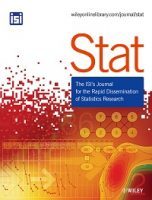# Lay abstract for Stat article: Anomaly detection of time series correlations via a novel Lie group structureEach week, we publish lay abstracts of new articles from our prestigious portfolio of journals in statistics. The aim is to highlight the latest research to a broader audience in an accessible format.

The article featured today is from Stat with the full article now available to read here.   (OPEN ACCESS)

, &  (2022). Anomaly detection of time series correlations via a novel Lie group structureStat111), e494. https://doi.org/10.1002/sta4.494

Correlations are one of the most fundamental quantities of study in the field of statistics and data science. This is generally attributed to the fact that they can be computed directly and are directly interpretable as the strength of the linear relationship between two random variables. Correlations take onscalar values between -1 and +1 where the closer the correlation is to either extreme represents a stronger linear relationship between the quantities. While this is generally accepted as a valid interpretation of correlation values, it has remained unclear as to how we measure the strength of a correlation. For instance, how much stronger is a correlation of 0.8 compared to one of 0.9, or 0.99?The main contribution of this article is in answering the above question, and by properly deriving a natural distance between correlations. At first glance it might seem unnecessary to ask such a question.After all, why cant one simply subtract one correlation value from another (e.g. the distance between 0.9 and 0.8 is simply 0.1)? The problem of this linear (i.e. Euclidean distance) arises when we try to perform statistics on a series of correlation values. For instance, say we have a series of correlation values c1,…,cN. We should be able to compute their average as well as the standard deviation of these values. This is where the interpretation of the linear distance fails, because it’s here that we can find standard deviation windowsσthat go outside the range of values(−1,1)representing the set of correlations. How can we address this problem?In this article we use the tools of differential geometry and group theory: their intersection of the two subjects being the study of Lie groups(pronounced “Lee”). The theory of Lie groups gives us the proper tools to characterize correlations in terms of a natural multiplication rule (derived from the matrix formalism for correlations), and to eventually give us an intrinsically defined notion of distance which by passes the issues involved with computing standard deviations in the Euclidean case.After developing this theoretical component characterizing correlations as a Lie group, we then use these tools to perform anomaly detection: finding instances of above average correlation using means and standard deviations of correlation values. Specifically we analyze correlations of stock data to identify instances in which stock prices are highly correlated to one another.